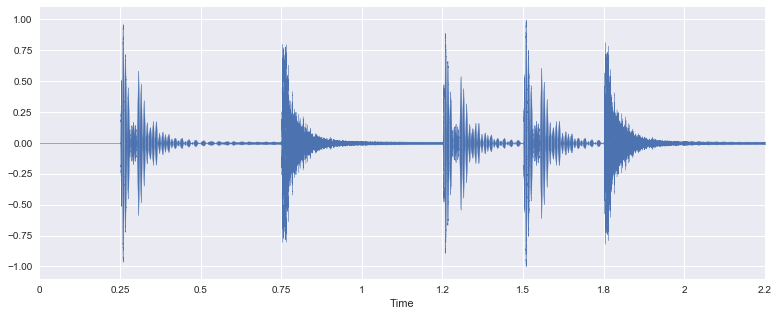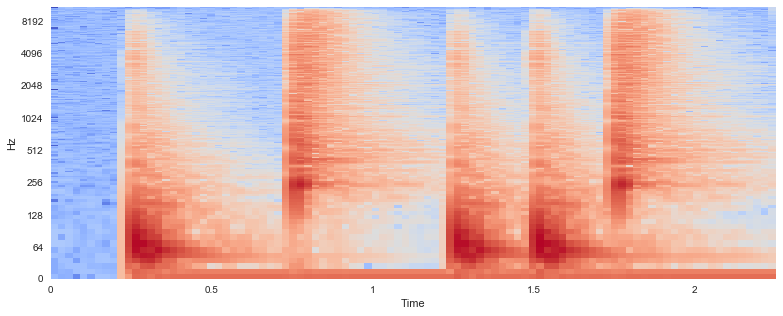In :
%matplotlib inline
import seaborn
import numpy, scipy, matplotlib.pyplot as plt, IPython.display as ipd
import librosa, librosa.display
plt.rcParams['figure.figsize'] = (13, 5)


# Understanding Audio Features through SonificationÂ¶

In this exercise notebook, we will segment, feature extract, and analyze audio files. Goals:

1. Detect onsets in an audio signal.
2. Segment the audio signal at each onset.
3. Compute features for each segment.
4. Gain intuition into the features by listening to each segment separately.

## Step 1: Retrieve AudioÂ¶

Load the audio file simple_loop.wav into an array.

In :
x, sr = librosa.load('audio/simple_loop.wav')


Show the sample rate:

In :
print sr

22050


Listen to the audio signal.

In :
ipd.Audio(x, rate=sr)

Out:

Display the audio signal.

In :
librosa.display.waveplot(x, sr=sr)

Out:
<matplotlib.collections.PolyCollection at 0x1153fed90>Compute the short-time Fourier transform:

In :
X = librosa.stft(x)


For display purposes, compute the log amplitude of the STFT:

In :
Xmag = librosa.logamplitude(X)


Display the spectrogram.

In :
# Play with the parameters, including x_axis and y_axis
librosa.display.specshow(Xmag, sr=sr, x_axis='time', y_axis='log')

Out:
<matplotlib.axes._subplots.AxesSubplot at 0x1152a9e90>## Step 2: Detect OnsetsÂ¶

Find the times, in seconds, when onsets occur in the audio signal.

In :
onset_frames = librosa.onset.onset_detect(x, sr=sr)
print onset_frames

[12 33 55 66 76]

In :
onset_times = librosa.frames_to_time(onset_frames, sr=sr)
print onset_times

[ 0.27863946  0.7662585   1.27709751  1.53251701  1.76471655]


Convert the onset frames into sample indices.

In :
onset_samples = librosa.frames_to_samples(onset_frames)
print onset_samples

[ 6144 16896 28160 33792 38912]


Play a "beep" at each onset.

In :
# Use the length parameter so the click track is the same length as the original signal
clicks = librosa.clicks(times=onset_times, length=len(x))

In :
# Play the click track "added to" the original signal
ipd.Audio(x+clicks, rate=sr)

Out:

## Step 3: Segment the AudioÂ¶

Save into an array, segments, 100-ms segments beginning at each onset.

In :
frame_sz = int(0.100*sr)
segments = numpy.array([x[i:i+frame_sz] for i in onset_samples])


Here is a function that adds 300 ms of silence onto the end of each segment and concatenates them into one signal.

Later, we will use this function to listen to each segment, perhaps sorted in a different order.

In :
def concatenate_segments(segments, sr=22050, pad_time=0.300):
padded_segments = [numpy.concatenate([segment, numpy.zeros(int(pad_time*sr))]) for segment in segments]

In :
concatenated_signal = concatenate_segments(segments, sr)


Listen to the newly concatenated signal.

In :
ipd.Audio(concatenated_signal, rate=sr)

Out:

## Step 4: Extract FeaturesÂ¶

For each segment, compute the zero crossing rate.

In :
zcrs = [sum(librosa.core.zero_crossings(segment)) for segment in segments]
print zcrs

[11, 570, 11, 10, 568]


Use argsort to find an index array, ind, such that segments[ind] is sorted by zero crossing rate.

In :
ind = numpy.argsort(zcrs)
print ind

[3 0 2 4 1]


Sort the segments by zero crossing rate, and concatenate the sorted segments.

In :
concatenated_signal = concatenate_segments(segments[ind], sr)


## Step 5: Listen to SegmentsÂ¶

Listen to the sorted segments. What do you hear?

In :
ipd.Audio(concatenated_signal, rate=sr)

Out:

## More ExercisesÂ¶

Repeat the steps above using other features from librosa.feature, e.g. rmse, spectral_centroid, spectral_bandwidth.

Repeat the steps above for other audio files:

In :
ls audio

c_strum.wav       conga_groove.wav  simple_loop.wav
clarinet_c6.wav   oboe_c6.wav       tone_440.wav